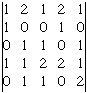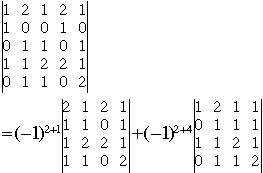Name: POH Who is asking: Other Level: All Question: 1) Determinants by expansion by minors. i) | 1 2 1 2 1 | | 1 0 0 1 0 | | 0 1 1 0 1 | | 1 1 2 2 1 | | 0 1 1 0 2 | 2) Solve the given system of equations by determinants.Evaluate the determinants by expansion by minors i) 2x + y + z = 6 x - 2y + 2z =10 3x - y - z = -2 ii) x + 2y -z =6 y - 2z - 3t = 5t 3x - 2y + t =2 2x + y +z -t =0 Thank for your help and can you show me the working. Thank you sir Hi, You can evaluate a determinant using minors along any row or column. Looking at your determinantI would expand along the second row in order to take advantage of the 3 zeros in that row. ThusNow you need to expand each of the 4x4 determinants. Again I would expand along the second row in each case. This will give you six, 3x3 determinants. Expanding each, using minirs along a row or column wil give 2x2 determinants which you can evaluate. Cheers, Harley Go to Math Central